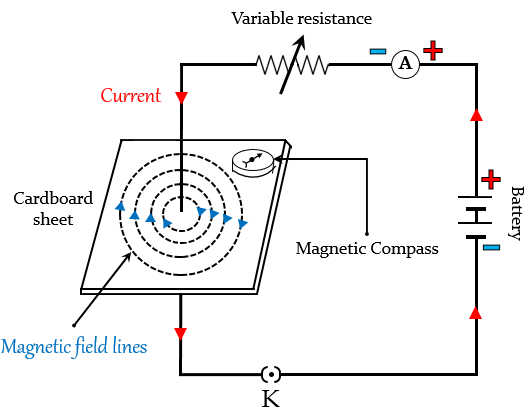# Draw the pattern of magnetic field lines produced around a current-carrying straight conductor passing perpendicularly through horizontal cardboard. State and apply the right-hand thumb rule to mark the direction of the field lines. How will the strength of the magnetic field change when the point where magnetic field is to be determined is moved away from the straight conductor ? Give reason to justify your answer.To mark the direction of magnetic field lines, we will use the Right-hand thumb rule.

According to this rule, if we hold the current-carrying conductor in the right hand in such a way that the thumb points towards the direction of the current, then the fingers wrapped around the conductor will give the direction of magnetic field lines.

The strength of the magnetic field will decrease/reduce when the point where the magnetic field is to be determined is moved away from the straight conductor, because the strength of the magnetic field is inversely proportional to the distance from the origin, $(B\propto \frac {1}{r})$.

Updated on: 10-Oct-2022

147 Views